››› Screenshot

THE SLIDE RULE OF SILICON DESIGN

Free Analog Circuit Simulation

Getting Started

A Simple Transistor Amplifier

Let us consider a simple transistor amplifier shown on figure below.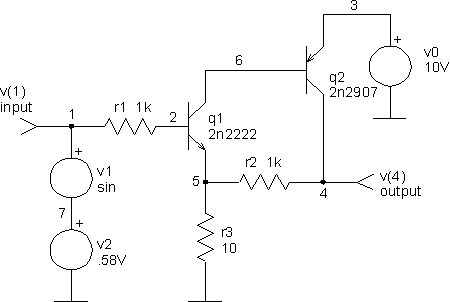First we have to describe the circuit in a *.cir file. The circuit netlist can be put together with some schematic editor or it can be written in a text editor. The netlist of the simple amplifier (amplifier.cir) is as follows:

Transistor amplifier
* this is amplifier.cir file
* input node 1
* output node 4
* voltage resources
v0 3 0 dc 10V
v1 1 7 dc 0V ac 1 sin 0 0.1V 10kHz
v2 7 0 dc 0.58V
* bipolar transistors
q1 6 2 5 2n2222
q2 4 6 3 2n2907
* resistors
r1 1 2 1k
r2 5 4 1k
r3 5 0 10
* model for a 2n2222 transistor
.model 2n2222 npn (is=19f bf=150 vaf=100 ikf=0.18 ise=50p
+ ne=2.5 br=7.5 var=6.4 ikr=12m isc=8.7p nc=1.2 rb=50 re=0.4
+ rc=0.3 cje=26p tf=0.5n cjc=11p tr=7n xtb=1.5 kf=0.032f af=1)
* model for a 2n2907 transistor
.model 2n2907 pnp (is=1.1P bf=200 nf=1.2 vaf=50 ikf=0.1 ise=13p
+ ne=1.9 br=6 rc=0.6 cje=23p vje=0.85 mje=1.25 tf=0.5n cjc=19p
+ vjc=0.5 mjc=0.2 tr=34n xtb=1.5)
.end

When the netlist of the circuit is written (in our case the amplifier.cir file) the circuit can be simulated. Run SpiceOpus. The SpiceOpus Command window will appear and the program is waiting for our first command with prompt:

SpiceOpus (c) 1 -> _

First we have to load the netlist of the circuit into the simulator. We can do this with source command:

SpiceOpus (c) 1 -> source amplifier.cir
SpiceOpus (c) 2 -> _

We perform a dc, transient and ac analyses with dc, tran and ac commands respectively. The voltage source v2 is swept from 0 to 1V in 5mV increments in the dc analysis. A transient analysis from 0 to 200us with a 10kHz sine wave input is performed by tran command. And the input frequency is swept from 1kHz to 10MHz with 25 points per decade in ac analysis:

SpiceOpus (c) 2 -> dc v2 0 1V 5mV
SpiceOpus (c) 3 -> tran 1us 200us
SpiceOpus (c) 4 -> ac dec 25 1kHz 100megHz
SpiceOpus (c) 5 -> _

The results are saved in a special data structures called plots. Our three analyses just created three new plots. We can change the current plot with a setplot command. The results of a particular analysis can be plotted by plot command.

SpiceOpus (c) 5 -> setplot
new      New plot
Current ac1   transistor amplifier (AC analysis)
tran1    transistor amplifier (Transient analysis)
dc1      transistor amplifier (DC transfer characteristic)
const    Constant values (constants)
SpiceOpus (c) 6 -> setplot dc1
SpiceOpus (c) 7 -> plot v(4) xlabel v(2)[V] ylabel Output[V]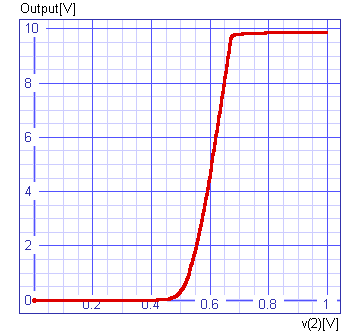SpiceOpus (c) 8 -> setplot tran1
SpiceOpus (c) 9 -> plot 10*v(1) v(4) xlabel t[s] ylabel '10*Input, Output [V]'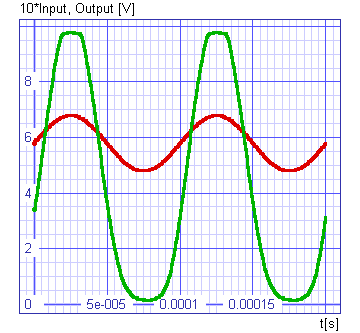SpiceOpus (c) 10 -> setplot ac1
SpiceOpus (c) 11 -> _

To plot phase in degrees (not in radians) the units variable has to be set. We can see the discontinuity of the phase when plotted. That occur because SpiceOpus calculates phase in an [-180°, 180°] interval.

SpiceOpus (c) 11 -> set units = degrees
SpiceOpus (c) 12 -> plot vdb(4) vp(4)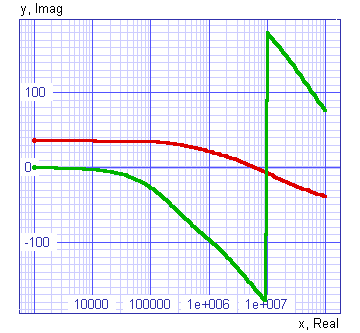SpiceOpus (c) 13 -> _

The discontinuity can be eliminated with defining a new vector called phase. The expression for it transfers all positive components for 360°.

SpiceOpus (c) 13 -> let phase = unwrap(vp(4))
SpiceOpus (c) 14 -> plot vdb(4) phase xlabel f[Hz] ylabel 'Magnitude[dB], Phase[deg]'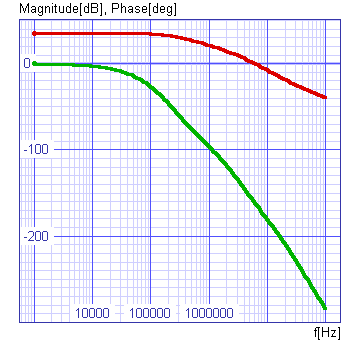SpiceOpus (c) 15 -> _

At the end we will delete all plots and free memory with destroy command and leave SpiceOpus with quit command.

SpiceOpus (c) 15 -> destroy all
SpiceOpus (c) 16 -> quit
Spice-3f4 done

The whole example with the commands in the .control block can be downloaded as amplifier_run.cir. You can start it by typing

SpiceOpus (c) 15 -> source amplifer_run.cir

at the SPICE OPUS command prompt.